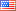# Revision history [back]

### replicate recursive sum

i have a function defined as: (cant post links or images so ill put the latex code)

 \begin{align} f(0) &= 1\\ f(n) &= 1 + \sum_{j=0}^{n-1}f(j) \text{ for every } n > 0 \end{align}



how can i replicate this function in sagemath using the sum function? i know it can be done in pure python with recursion but then whats the point of sagemath and symbolic calculation?

what ive tried so far is:

 f(x) = 1 if x == 0 else sum(1+f(j-1), j, 0, x-1) 

but i get the following error:

 NameError: name 'f' is not defined 

now i know its not possible to use recursion on a variable like this but there must be some tricky way to go about doing that (i hope so and thats why im here)

### replicate recursive sum

i have a function defined as: (cant post links or images so ill put the latex code)as

 \begin{align} f(0) &= 1\\ f(n) &= 1 + \sum_{j=0}^{n-1}f(j) \text{ for every } n > 0 \end{align}



https ://imgur.com/a/SfF2Edl.png

how can i replicate this function in sagemath using the sum function? i know it can be done in pure python with recursion but then whats the point of sagemath and symbolic calculation?

what ive tried so far is:

 f(x) = 1 if x == 0 else sum(1+f(j-1), j, 0, x-1) 

but i get the following error:

 NameError: name 'f' is not defined 

now i know its not possible to use recursion on a variable like this but there must be some tricky way to go about doing that (i hope so and thats why im here)3 NoneJohn Palmieri8131 ●19 ●69 ●177 http://www.math.washin...

### replicate recursive sum

i have a function defined as

https ://imgur.com/a/SfF2Edl.pnghttps://imgur.com/a/SfF2Edl.png

how can i replicate this function in sagemath using the sum function? i know it can be done in pure python with recursion but then whats the point of sagemath and symbolic calculation?

what ive tried so far is:

 f(x) = 1 if x == 0 else sum(1+f(j-1), j, 0, x-1) 

but i get the following error:

 NameError: name 'f' is not defined 

now i know its not possible to use recursion on a variable like this but there must be some tricky way to go about doing that (i hope so and thats why im here)4 None

### replicate recursive sum

i have a function defined as

https://imgur.com/a/SfF2Edl.pnghow can i replicate this function in sagemath using the sum function? i know it can be done in pure python with recursion but then whats the point of sagemath and symbolic calculation?

what ive tried so far is:

 f(x) = 1 if x == 0 else sum(1+f(j-1), j, 0, x-1) 

but i get the following error:

 NameError: name 'f' is not defined 

now i know its not possible to use recursion on a variable like this but there must be some tricky way to go about doing that (i hope so and thats why im here)